# Selina Solutions Concise Maths Class 7 Chapter 5: Exponents (Including Laws of Exponents) Exercise 5B

Selina Solutions Concise Maths Class 7 Chapter 5 Exponents (Including Laws of Exponents) Exercise 5B explains the laws of exponents and more about indices. Product Law, Quotient Law and Power law are the main laws, which are discussed in brief under this exercise. The solutions PDF helps students analyse the areas of weaknesses and work on them for further progress. The solutions PDF can be referred by the students while solving textbook problems to clear doubts instantly. Selina Solutions Concise Maths Class 7 Chapter 5 Exponents (Including Laws of Exponents) Exercise 5B PDF links are given below for free download.

## Selina Solutions Concise Maths Class 7 Chapter 5: Exponents (Including Laws of Exponents) Exercise 5B Download PDF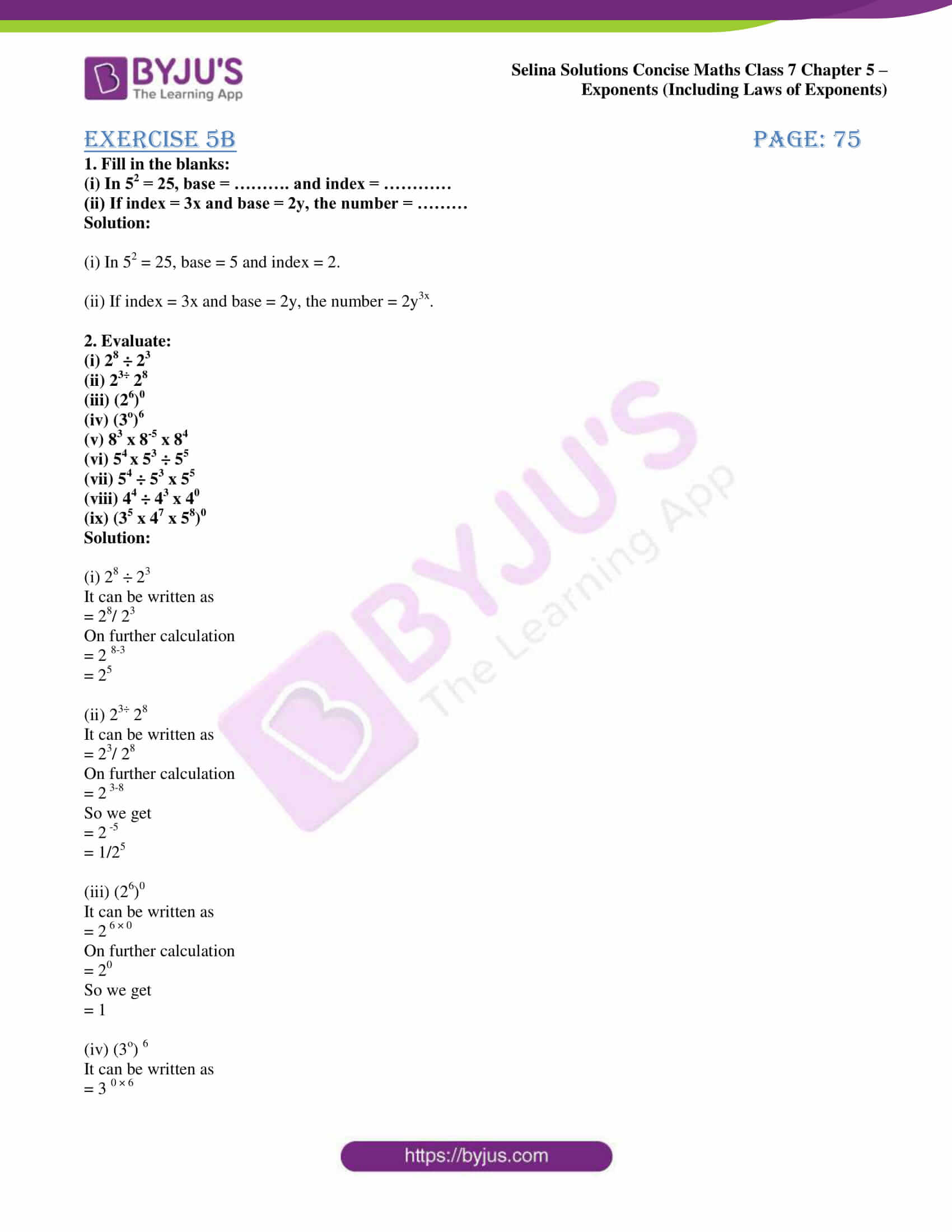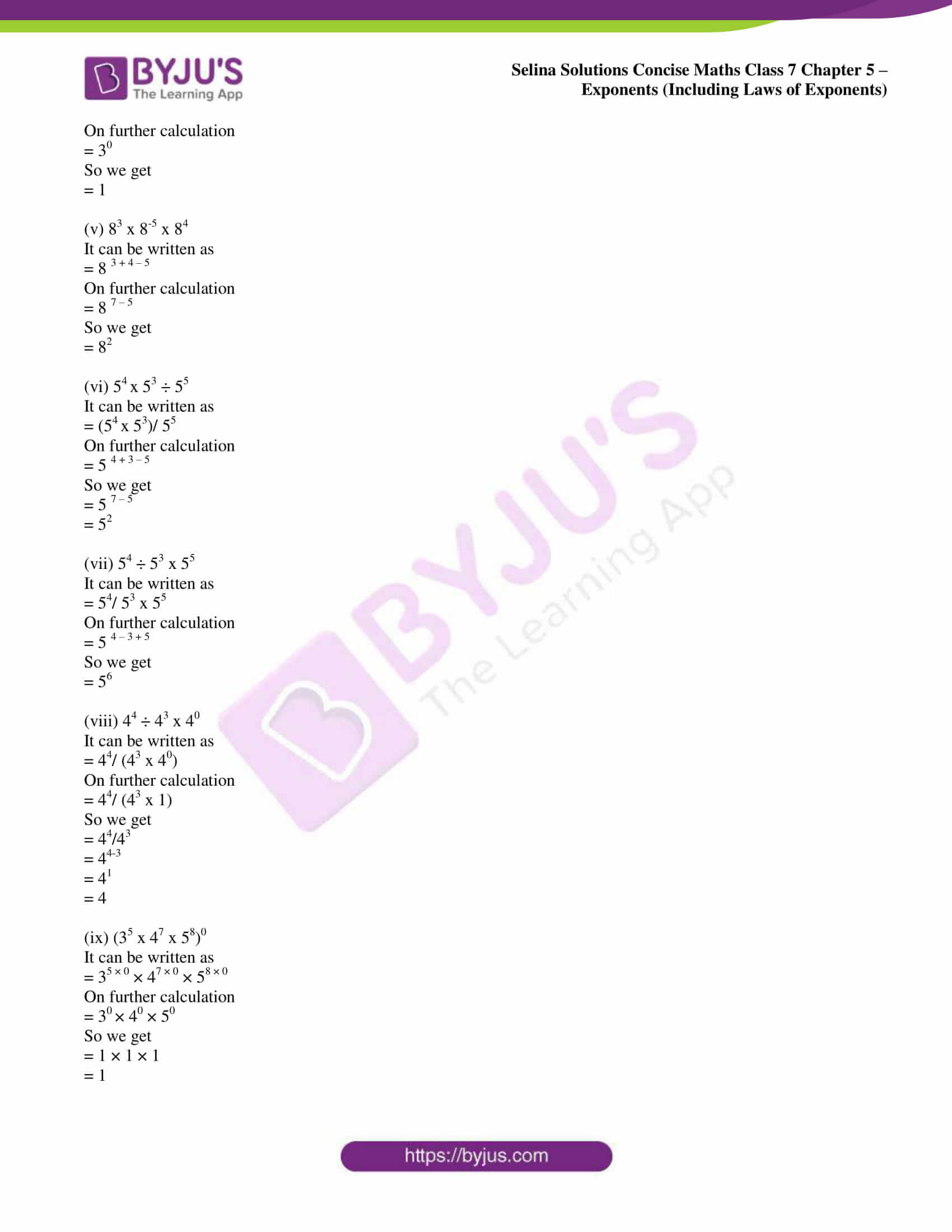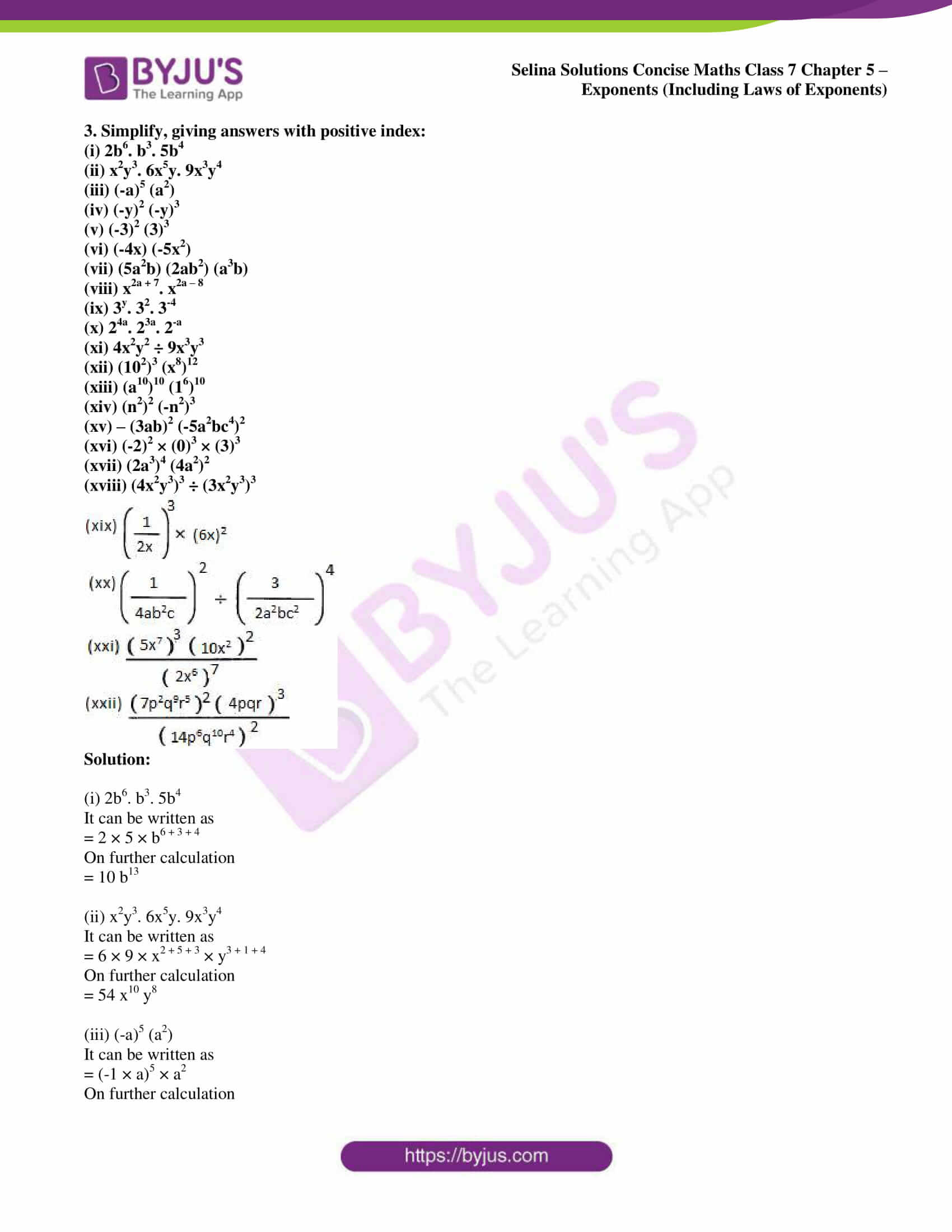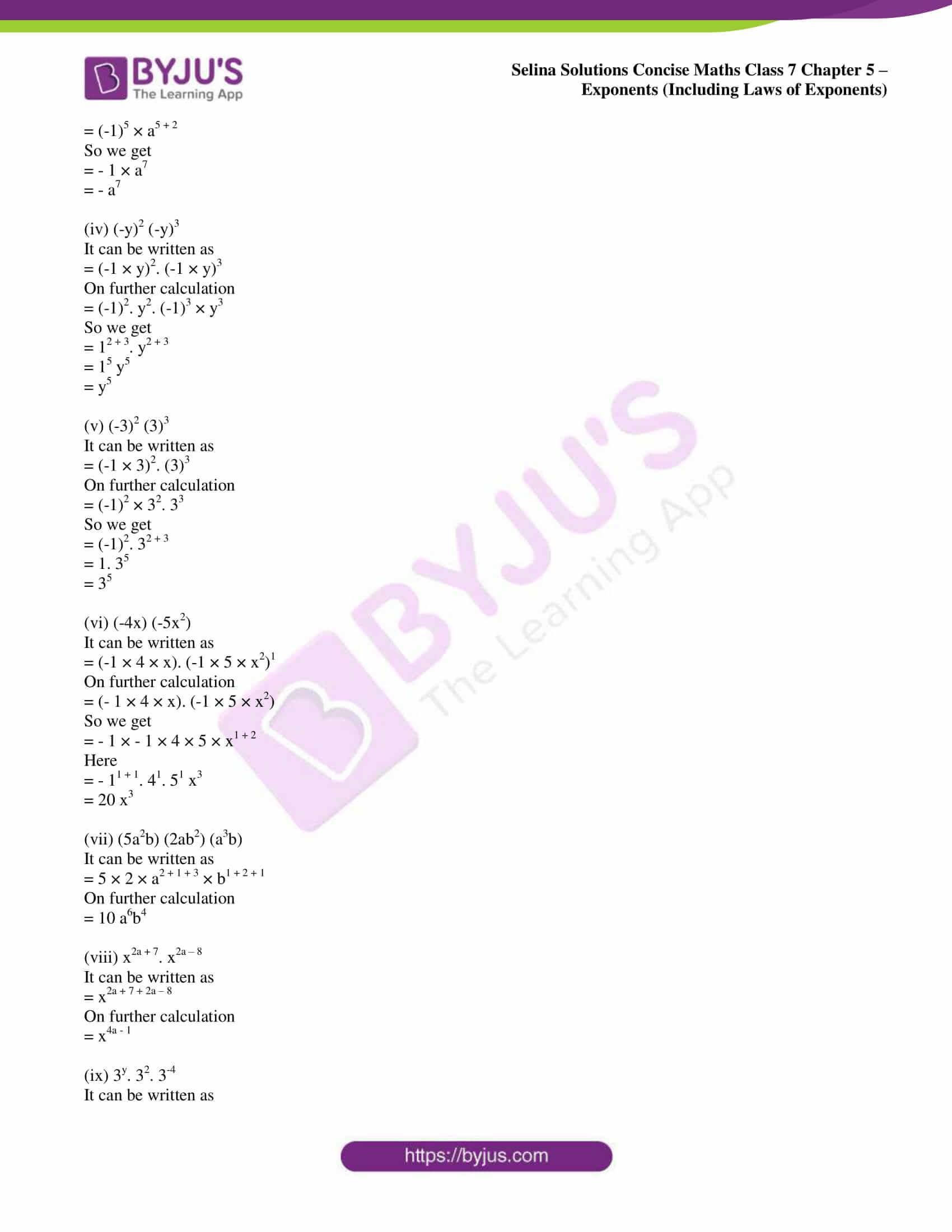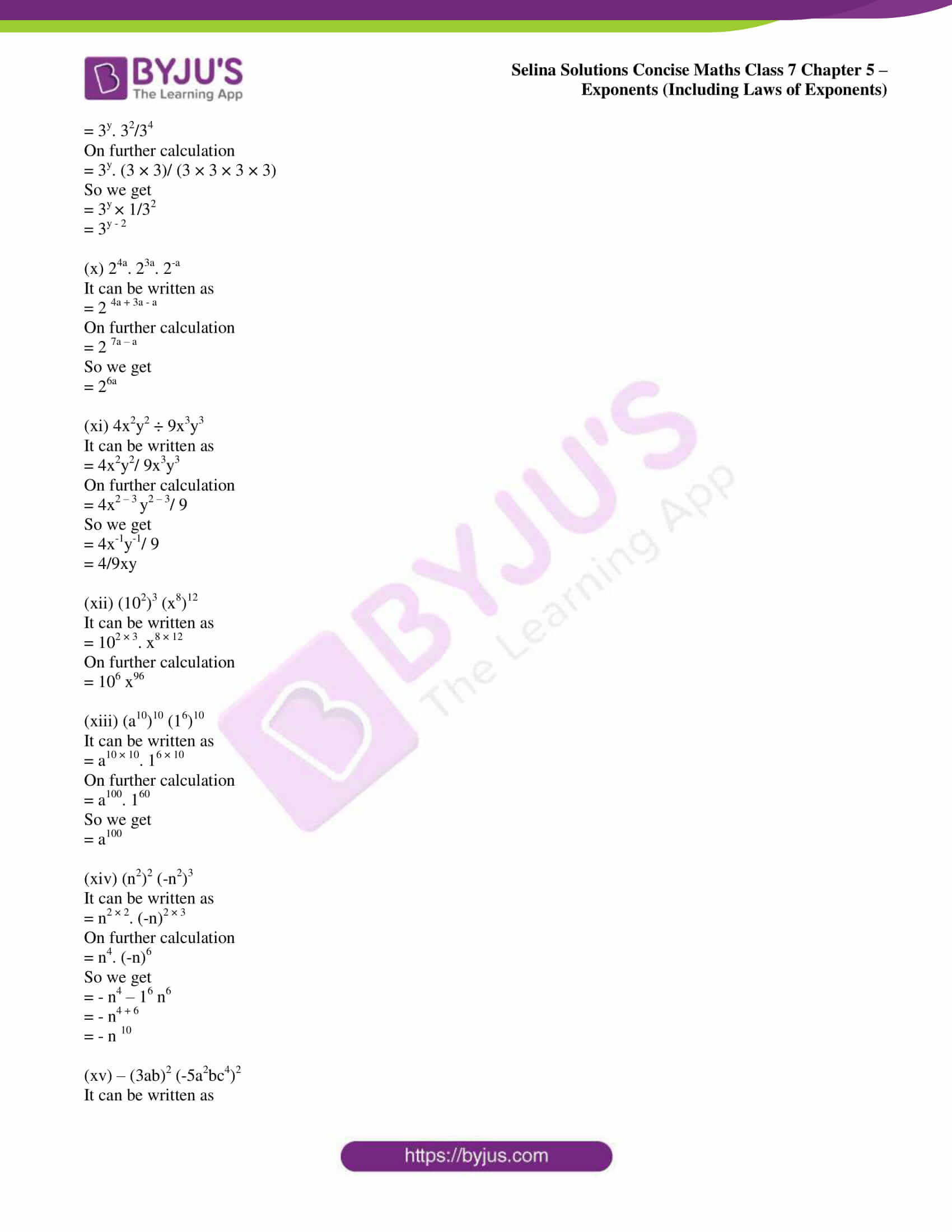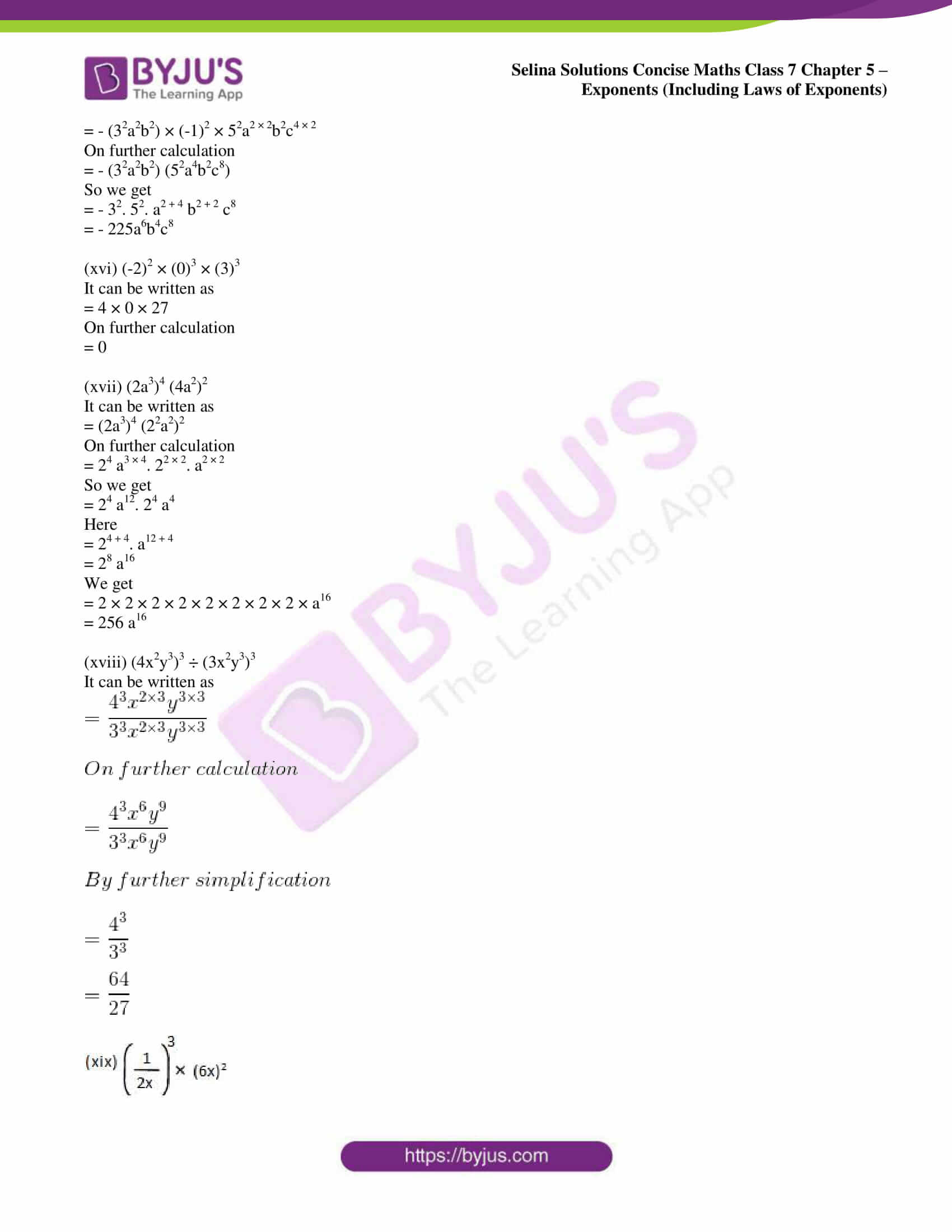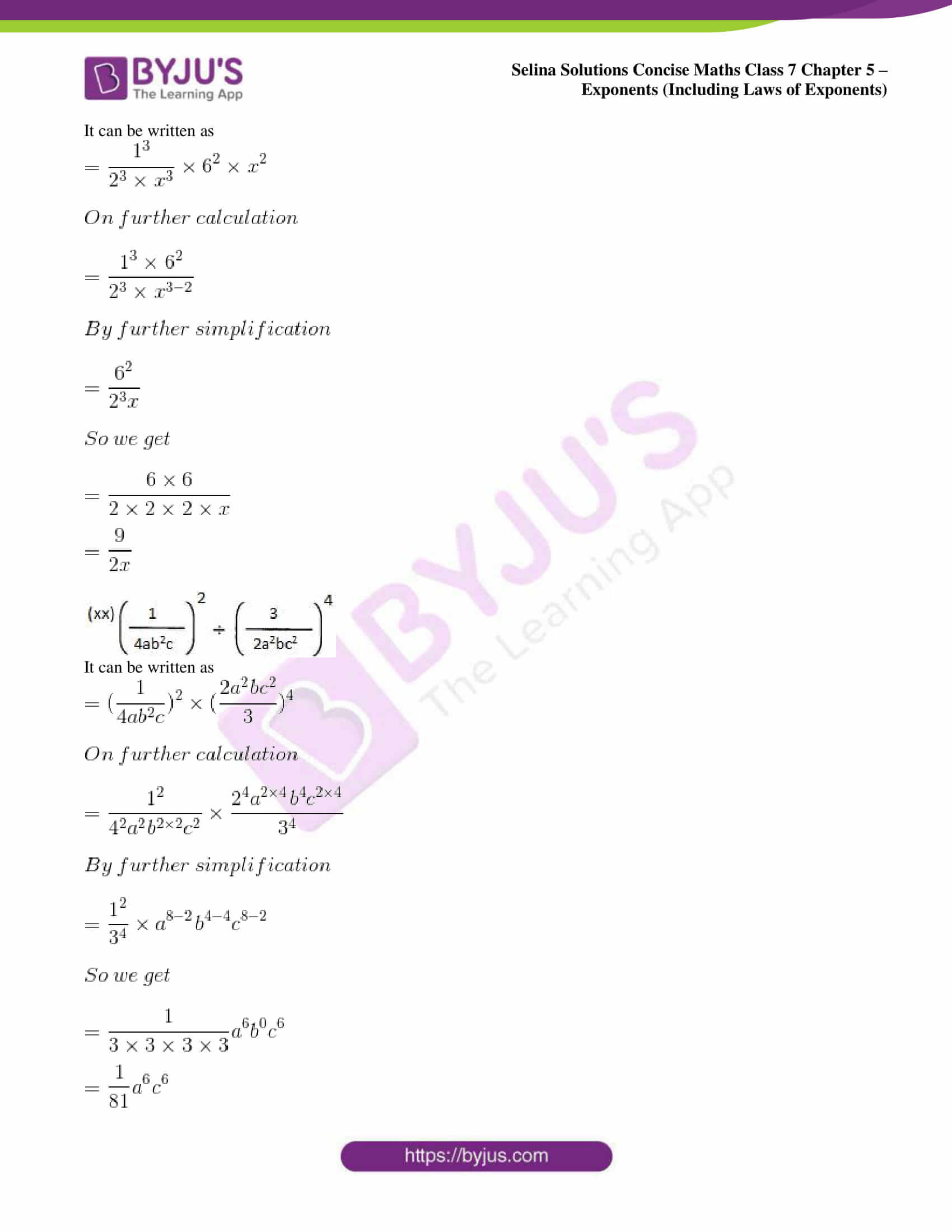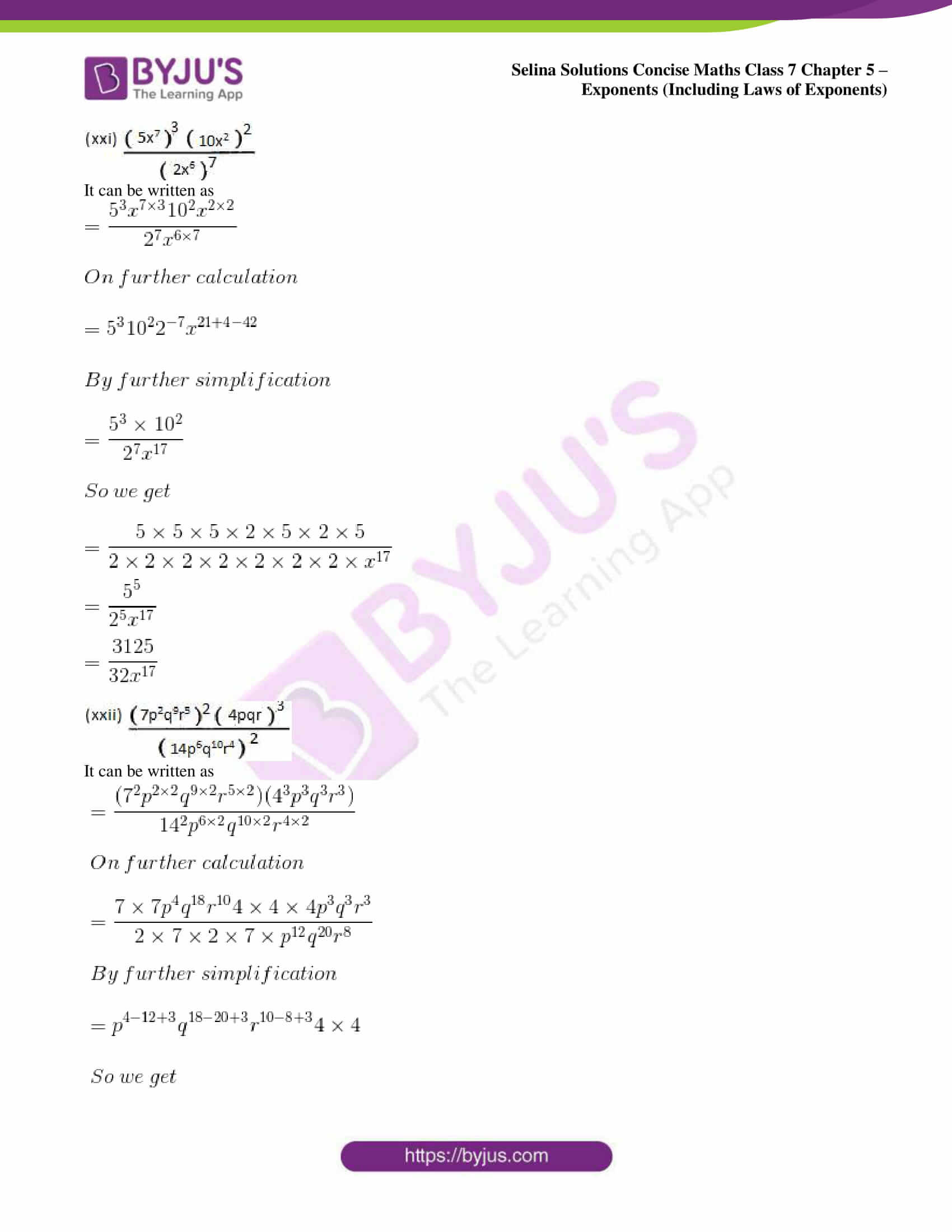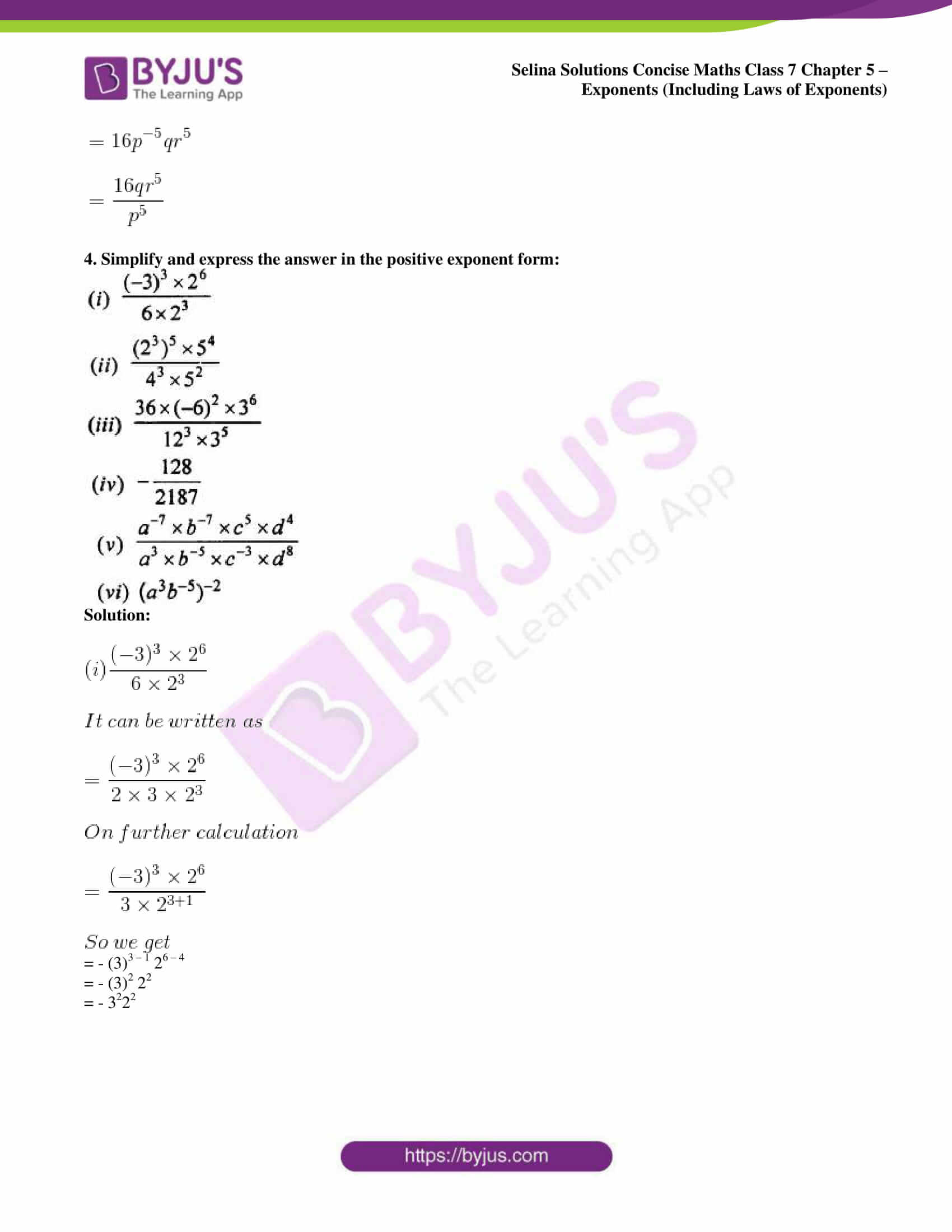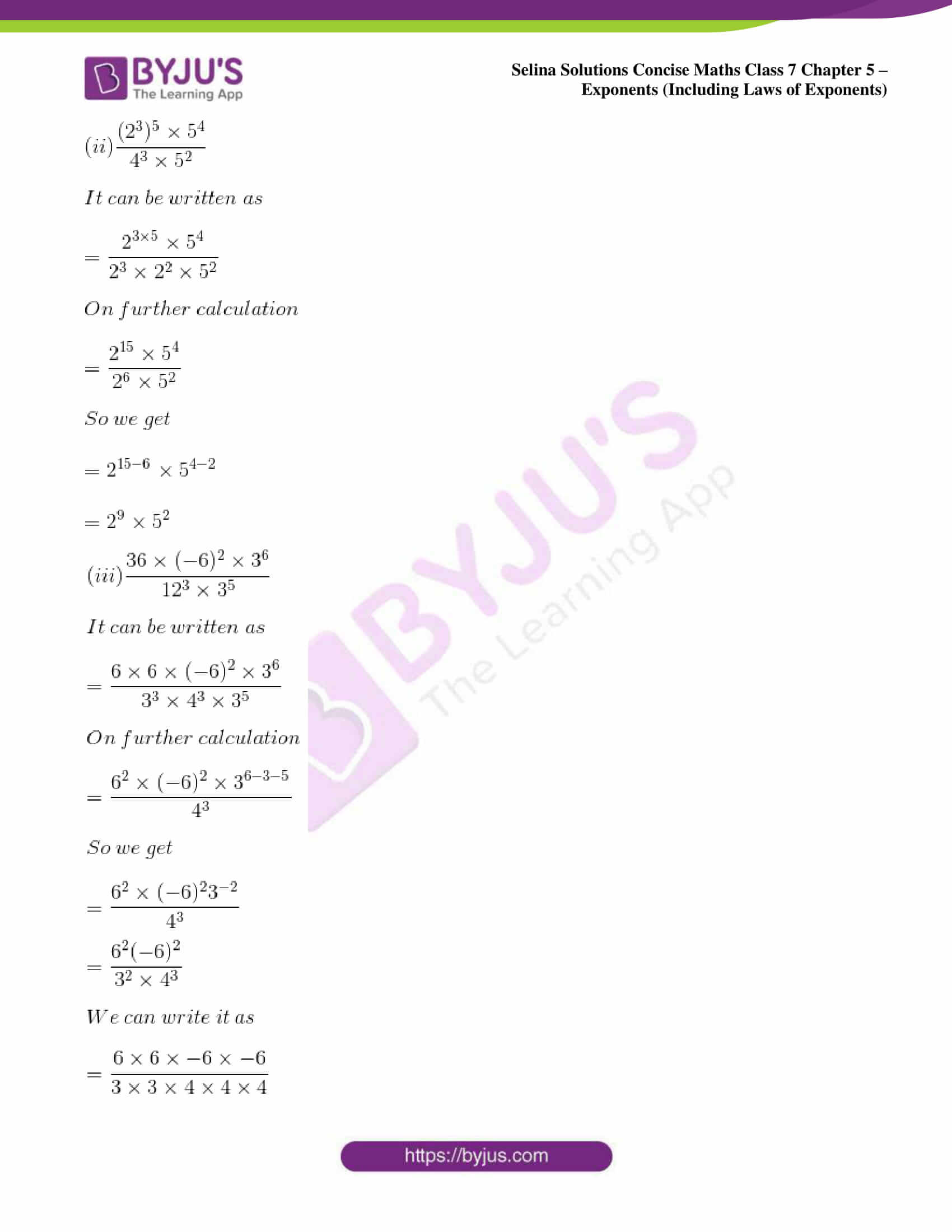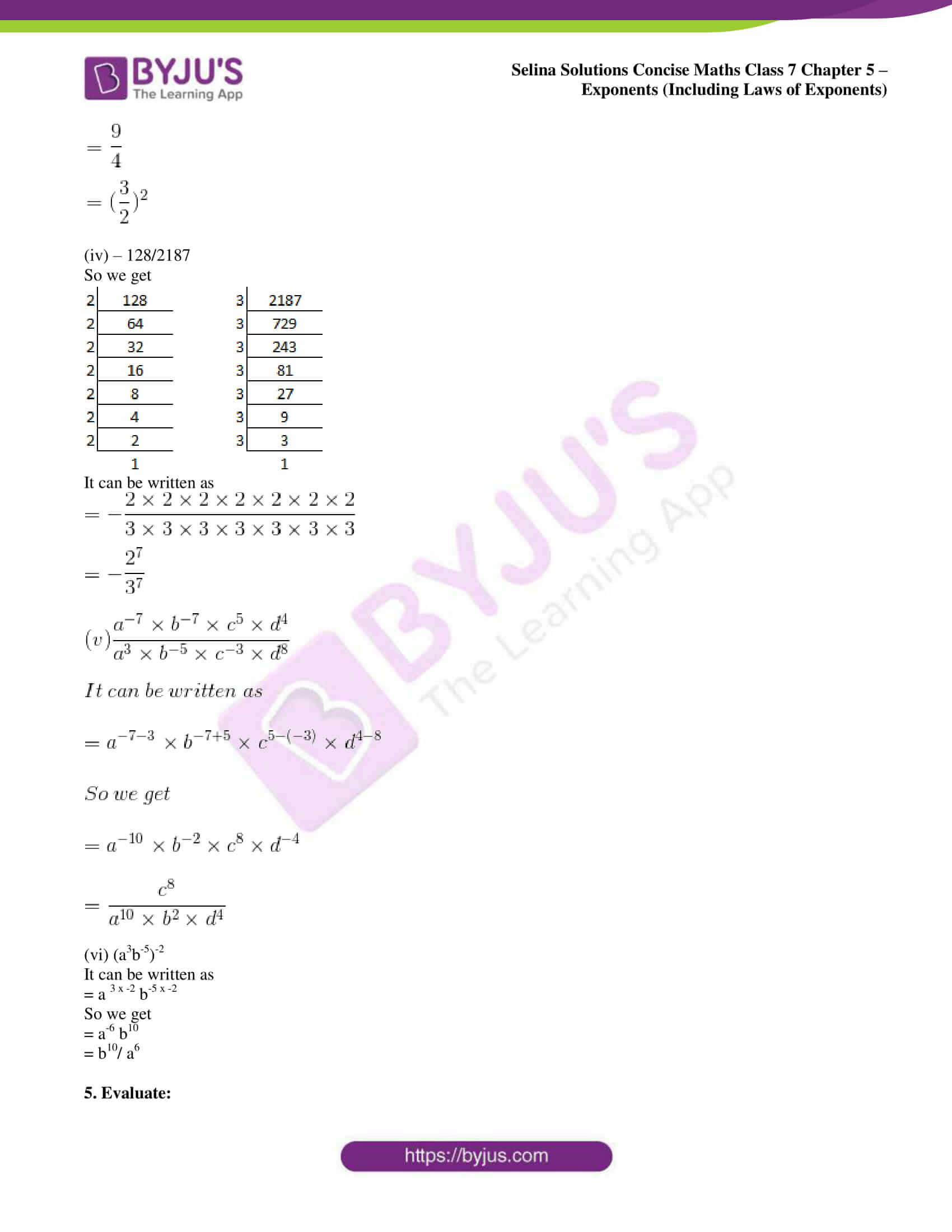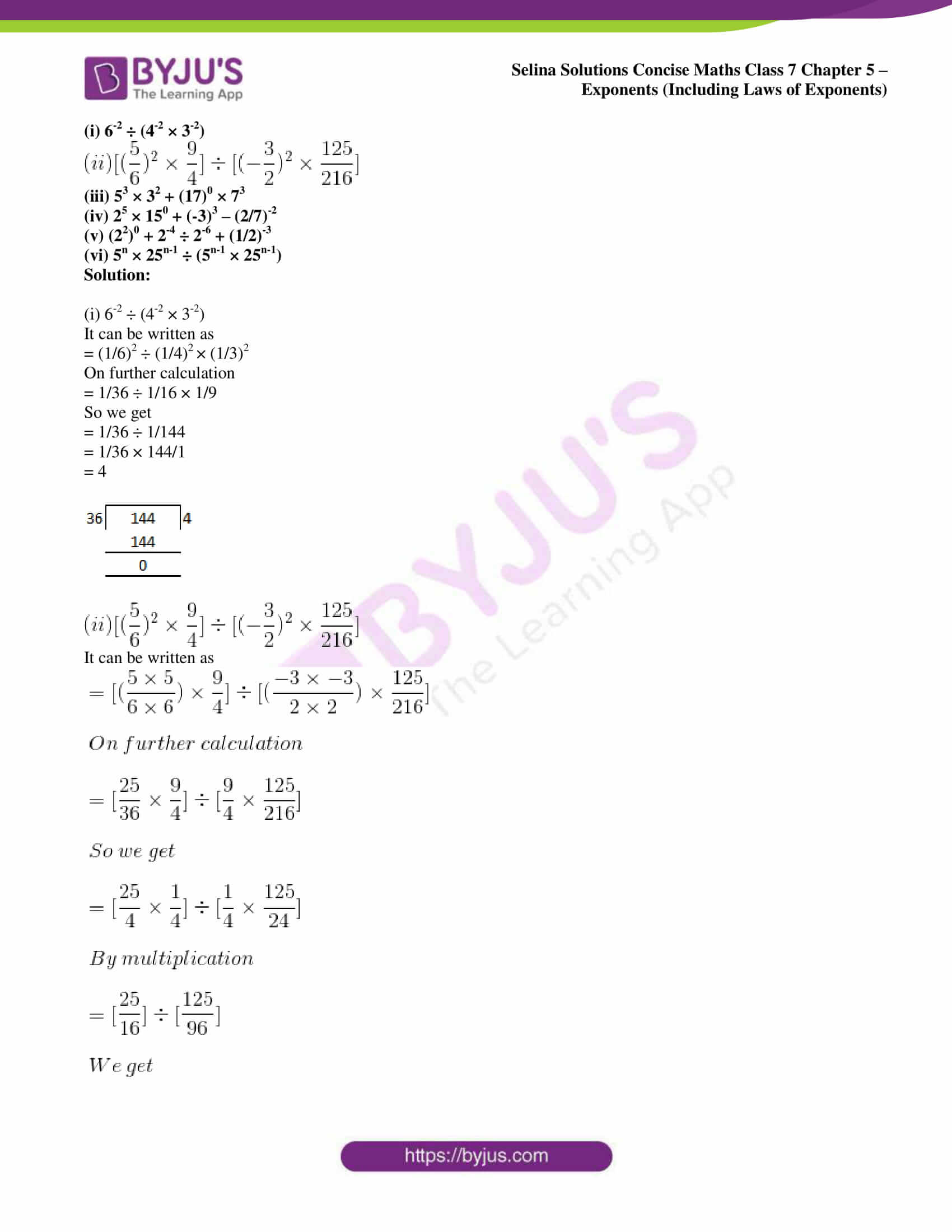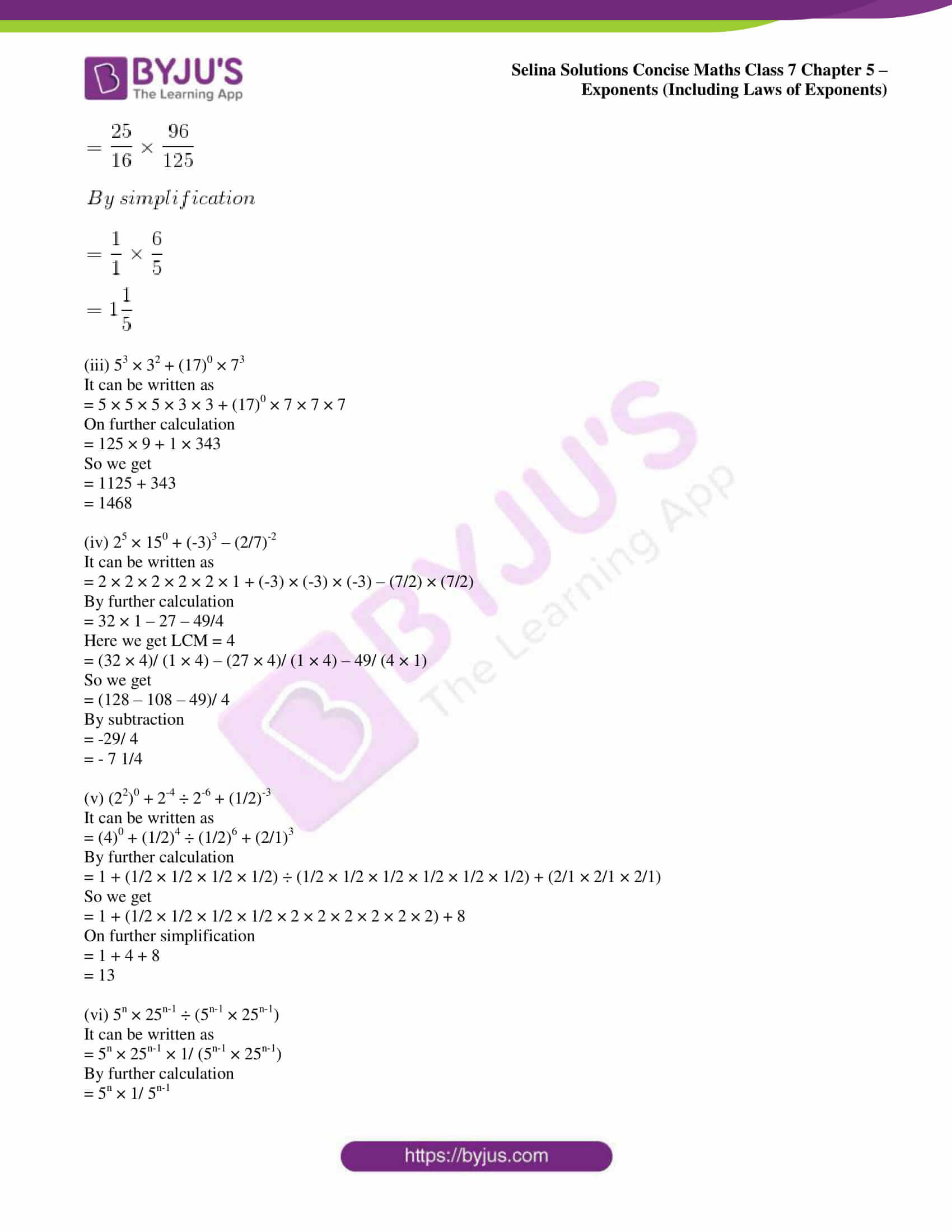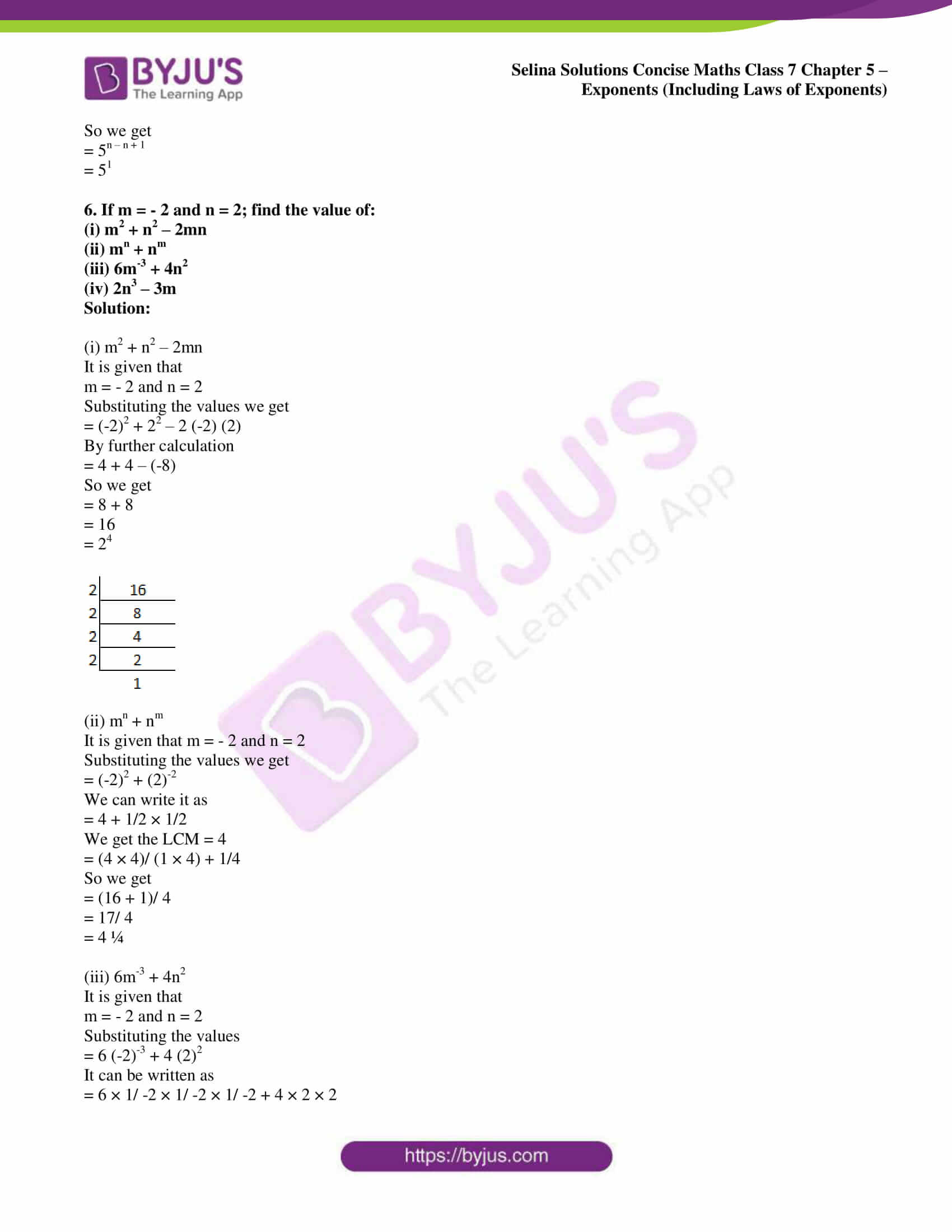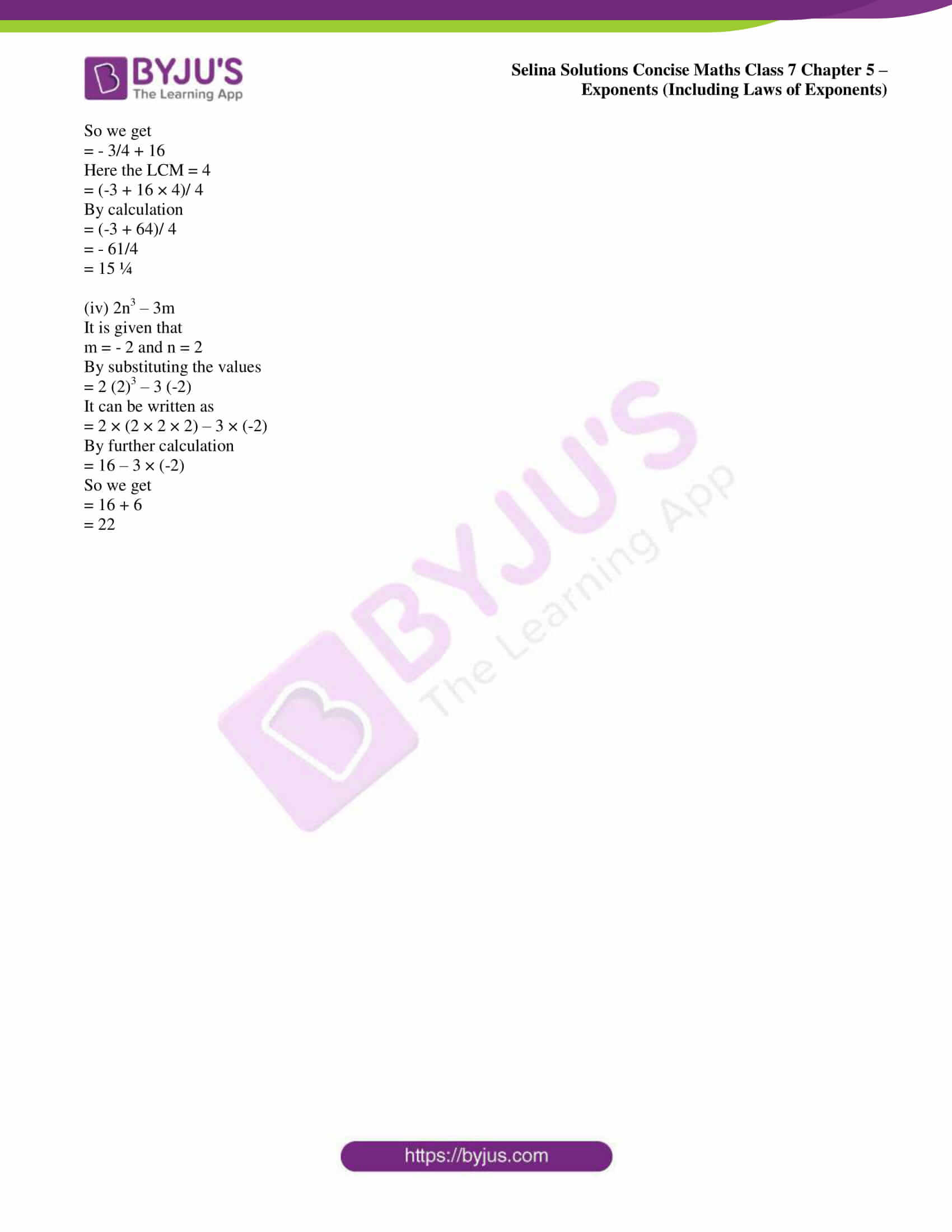### Access Selina Solutions Concise Maths Class 7 Chapter 5: Exponents (Including Laws of Exponents) Exercise 5B

1. Fill in the blanks:

(i) In 52 = 25, base = ………. and index = …………

(ii) If index = 3x and base = 2y, the number = ………

Solution:

(i) In 52 = 25, base = 5 and index = 2.

(ii) If index = 3x and base = 2y, the number = 2y3x.

2. Evaluate:

(i) 28 ÷ 23
(ii) 2 28
(iii) (26)0
(iv) (3o)6
(v) 83 x 8-5 x 84
(vi) 5x 53 ÷ 55
(vii) 54 ÷ 53 x 55
(viii) 44 ÷ 43 x 40
(ix) (35 x 47 x 58)0

Solution:

(i) 28 ÷ 23

It can be written as

= 28/ 23

On further calculation

= 2 8-3

= 25

(ii) 2 28

It can be written as

= 23/ 28

On further calculation

= 2 3-8

So we get

= 2 -5

= 1/25

(iii) (26)0

It can be written as

= 2 6 × 0

On further calculation

= 20

So we get

= 1

(iv) (3o) 6

It can be written as

= 3 0 × 6

On further calculation

= 30

So we get

= 1

(v) 83 x 8-5 x 84

It can be written as

= 8 3 + 4 – 5

On further calculation

= 8 7 – 5

So we get

= 82

(vi) 5x 53 ÷ 55

It can be written as

= (5x 53)/ 55

On further calculation

= 5 4 + 3 – 5

So we get

= 5 7 – 5

= 52

(vii) 54 ÷ 53 x 55

It can be written as

= 54/ 53 x 55

On further calculation

= 5 4 – 3 + 5

So we get

= 56

(viii) 44 ÷ 43 x 40

It can be written as

= 44/ (43 x 40)

On further calculation

= 44/ (43 x 1)

So we get

= 44/43

= 44-3

= 41

= 4

(ix) (35 x 47 x 58)0

It can be written as

= 35 × 0 × 47 × 0 × 58 × 0

On further calculation

= 30 × 40 × 50

So we get

= 1 × 1 × 1

= 1

3. Simplify, giving answers with positive index:

(i) 2b6. b3. 5b4

(ii) x2y3. 6x5y. 9x3y4

(iii) (-a)5 (a2)

(iv) (-y)2 (-y)3

(v) (-3)2 (3)3

(vi) (-4x) (-5x2)

(vii) (5a2b) (2ab2) (a3b)

(viii) x2a + 7. x2a – 8

(ix) 3y. 32. 3-4

(x) 24a. 23a. 2-a

(xi) 4x2y2 ÷ 9x3y3

(xii) (102)3 (x8)12

(xiii) (a10)10 (16)10

(xiv) (n2)2 (-n2)3

(xv) – (3ab)2 (-5a2bc4)2

(xvi) (-2)2 × (0)3 × (3)3

(xvii) (2a3)4 (4a2)2

(xviii) (4x2y3)3 ÷ (3x2y3)3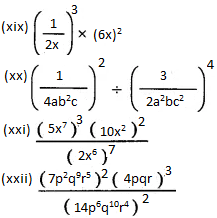Solution:

(i) 2b6. b3. 5b4

It can be written as

= 2 × 5 × b6 + 3 + 4

On further calculation

= 10 b13

(ii) x2y3. 6x5y. 9x3y4

It can be written as

= 6 × 9 × x2 + 5 + 3 × y3 + 1 + 4

On further calculation

= 54 x10 y8

(iii) (-a)5 (a2)

It can be written as

= (-1 × a)5 × a2

On further calculation

= (-1)5 × a5 + 2

So we get

= – 1 × a7

= – a7

(iv) (-y)2 (-y)3

It can be written as

= (-1 × y)2. (-1 × y)3

On further calculation

= (-1)2. y2. (-1)3 × y3

So we get

= 12 + 3. y2 + 3

= 15 y5

= y5

(v) (-3)2 (3)3

It can be written as

= (-1 × 3)2. (3)3

On further calculation

= (-1)2 × 32. 33

So we get

= (-1)2. 32 + 3

= 1. 35

= 35

(vi) (-4x) (-5x2)

It can be written as

= (-1 × 4 × x). (-1 × 5 × x2)1

On further calculation

= (- 1 × 4 × x). (-1 × 5 × x2)

So we get

= – 1 × – 1 × 4 × 5 × x1 + 2

Here

= – 11 + 1. 41. 51 x3

= 20 x3

(vii) (5a2b) (2ab2) (a3b)

It can be written as

= 5 × 2 × a2 + 1 + 3 × b1 + 2 + 1

On further calculation

= 10 a6b4

(viii) x2a + 7. x2a – 8

It can be written as

= x2a + 7 + 2a – 8

On further calculation

= x4a – 1

(ix) 3y. 32. 3-4

It can be written as

= 3y. 32/34

On further calculation

= 3y. (3 × 3)/ (3 × 3 × 3 × 3)

So we get

= 3y × 1/32

= 3y – 2

(x) 24a. 23a. 2-a

It can be written as

= 2 4a + 3a – a

On further calculation

= 2 7a – a

So we get

= 26a

(xi) 4x2y2 ÷ 9x3y3

It can be written as

= 4x2y2/ 9x3y3

On further calculation

= 4x2 – 3 y2 – 3/ 9

So we get

= 4x-1y-1/ 9

= 4/9xy

(xii) (102)3 (x8)12

It can be written as

= 102 × 3. x8 × 12

On further calculation

= 106 x96

(xiii) (a10)10 (16)10

It can be written as

= a10 × 10. 16 × 10

On further calculation

= a100. 160

So we get

= a100

(xiv) (n2)2 (-n2)3

It can be written as

= n2 × 2. (-n)2 × 3

On further calculation

= n4. (-n)6

So we get

= – n4 – 16 n6

= – n4 + 6

= – n 10

(xv) – (3ab)2 (-5a2bc4)2

It can be written as

= – (32a2b2) × (-1)2 × 52a2 × 2b2c4 × 2

On further calculation

= – (32a2b2) (52a4b2c8)

So we get

= – 32. 52. a2 + 4 b2 + 2 c8

= – 225a6b4c8

(xvi) (-2)2 × (0)3 × (3)3

It can be written as

= 4 × 0 × 27

On further calculation

= 0

(xvii) (2a3)4 (4a2)2

It can be written as

= (2a3)4 (22a2)2

On further calculation

= 24 a3 × 4. 22 × 2. a2 × 2

So we get

= 24 a12. 24 a4

Here

= 24 + 4. a12 + 4

= 28 a16

We get

= 2 × 2 × 2 × 2 × 2 × 2 × 2 × 2 × a16

= 256 a16

(xviii) (4x2y3)3 ÷ (3x2y3)3

It can be written as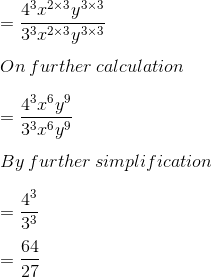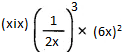It can be written as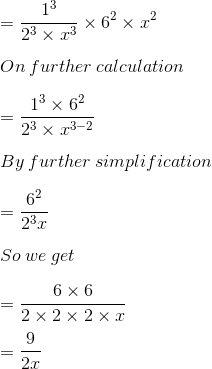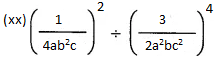It can be written as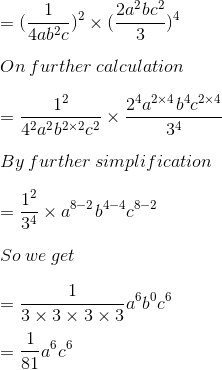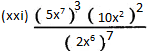It can be written as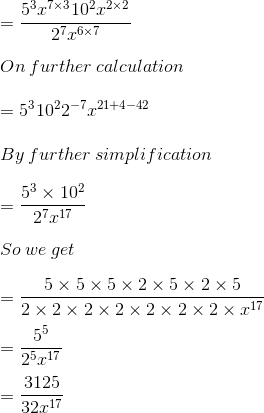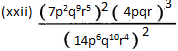It can be written as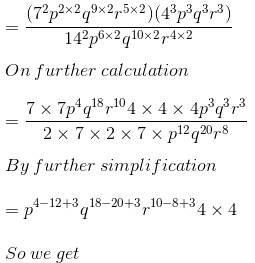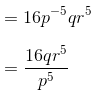4. Simplify and express the answer in the positive exponent form: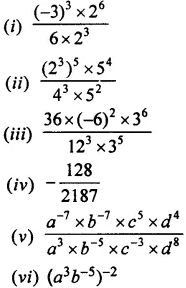Solution: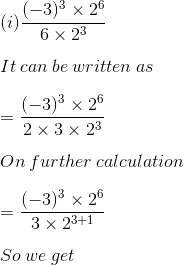= – (3)3 – 1 26 – 4

= – (3)2 22

= – 3222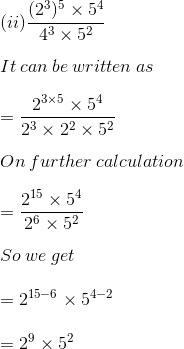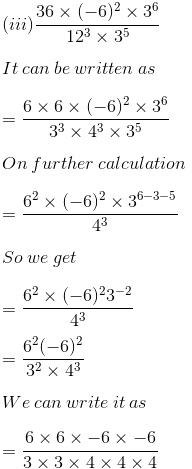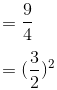(iv) – 128/2187

So we get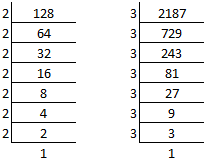It can be written as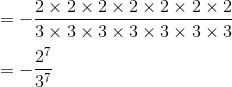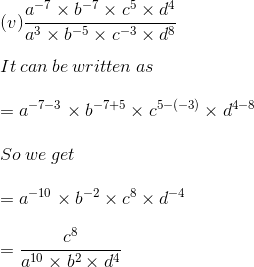(vi) (a3b-5)-2

It can be written as

= a 3 x -2 b-5 x -2

So we get

= a-6 b10

= b10/ a6

5. Evaluate:

(i) 6-2 ÷ (4-2 × 3-2)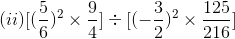(iii) 53 × 32 + (17)0 × 73

(iv) 25 × 150 + (-3)3 – (2/7)-2

(v) (22)0 + 2-4 ÷ 2-6 + (1/2)-3

(vi) 5n × 25n-1 ÷ (5n-1 × 25n-1)

Solution:

(i) 6-2 ÷ (4-2 × 3-2)

It can be written as

= (1/6)2 ÷ (1/4)2 × (1/3)2

On further calculation

= 1/36 ÷ 1/16 × 1/9

So we get

= 1/36 ÷ 1/144

= 1/36 × 144/1

= 4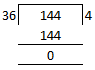It can be written as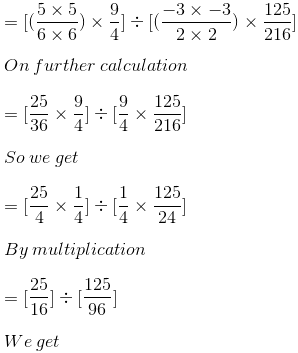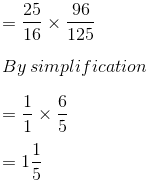(iii) 53 × 32 + (17)0 × 73

It can be written as

= 5 × 5 × 5 × 3 × 3 + (17)0 × 7 × 7 × 7

On further calculation

= 125 × 9 + 1 × 343

So we get

= 1125 + 343

= 1468

(iv) 25 × 150 + (-3)3 – (2/7)-2

It can be written as

= 2 × 2 × 2 × 2 × 2 × 1 + (-3) × (-3) × (-3) – (7/2) × (7/2)

By further calculation

= 32 × 1 – 27 – 49/4

Here we get LCM = 4

= (32 × 4)/ (1 × 4) – (27 × 4)/ (1 × 4) – 49/ (4 × 1)

So we get

= (128 – 108 – 49)/ 4

By subtraction

= -29/ 4

= – 7 1/4

(v) (22)0 + 2-4 ÷ 2-6 + (1/2)-3

It can be written as

= (4)0 + (1/2)4 ÷ (1/2)6 + (2/1)3

By further calculation

= 1 + (1/2 × 1/2 × 1/2 × 1/2) ÷ (1/2 × 1/2 × 1/2 × 1/2 × 1/2 × 1/2) + (2/1 × 2/1 × 2/1)

So we get

= 1 + (1/2 × 1/2 × 1/2 × 1/2 × 2 × 2 × 2 × 2 × 2 × 2) + 8

On further simplification

= 1 + 4 + 8

= 13

(vi) 5n × 25n-1 ÷ (5n-1 × 25n-1)

It can be written as

= 5n × 25n-1 × 1/ (5n-1 × 25n-1)

By further calculation

= 5n × 1/ 5n-1

So we get

= 5n – n + 1

= 51

6. If m = – 2 and n = 2; find the value of:

(i) m2 + n2 – 2mn

(ii) mn + nm

(iii) 6m-3 + 4n2

(iv) 2n3 – 3m

Solution:

(i) m2 + n2 – 2mn

It is given that

m = – 2 and n = 2

Substituting the values we get

= (-2)2 + 22 – 2 (-2) (2)

By further calculation

= 4 + 4 – (-8)

So we get

= 8 + 8

= 16

= 24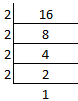(ii) mn + nm

It is given that m = – 2 and n = 2

Substituting the values we get

= (-2)2 + (2)-2

We can write it as

= 4 + 1/2 × 1/2

We get the LCM = 4

= (4 × 4)/ (1 × 4) + 1/4

So we get

= (16 + 1)/ 4

= 17/ 4

= 4 ¼

(iii) 6m-3 + 4n2

It is given that

m = – 2 and n = 2

Substituting the values

= 6 (-2)-3 + 4 (2)2

It can be written as

= 6 × 1/ -2 × 1/ -2 × 1/ -2 + 4 × 2 × 2

So we get

= – 3/4 + 16

Here the LCM = 4

= (-3 + 16 × 4)/ 4

By calculation

= (-3 + 64)/ 4

= – 61/4

= 15 ¼

(iv) 2n3 – 3m

It is given that

m = – 2 and n = 2

By substituting the values

= 2 (2)3 – 3 (-2)

It can be written as

= 2 × (2 × 2 × 2) – 3 × (-2)

By further calculation

= 16 – 3 × (-2)

So we get

= 16 + 6

= 22